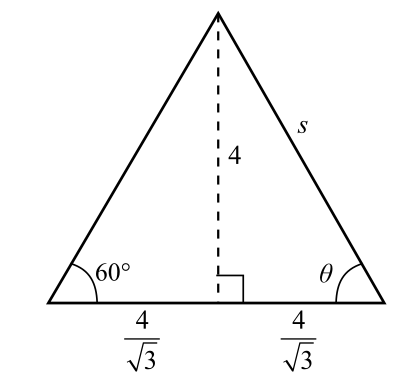Chapter 8.1, Problem 36E### Calculus: An Applied Approach (Min...

10th Edition
Ron Larson
ISBN: 9781305860919

#### Solutions

Chapter
Section### Calculus: An Applied Approach (Min...

10th Edition
Ron Larson
ISBN: 9781305860919
Textbook Problem
1 views

# Analyzing Triangles In Exercises 33-40, solve the triangle for the indicated side and/or angle.To determine

To calculate: The value of side s and angle θ in a triangle provided below.Explanation

Given Information:

The provided triangle is shown below,

Formula used:

Pythagorean theorem for any right triangle is such that c2=a2+b2, where a, b are the two perpendicular sides of a triangle and c is the length of hypotenuse.

In an isosceles triangle, two sides are equal and its two angles opposite the sides are equal.

In an equilateral triangle, each side is equal and each angle is 60°. In this triangle, the altitude bisects it base.

Calculation:

From the observation of the triangle, the altitude bisects the side into two equal parts of 43. It means that the triangle is isosceles.

Consider the sides of right triangle, 4, 43 and s

The value of side a is 4, base b is 43 and hypotenuse c is s.

Substitute a=4, b=43 and c=s in the equation c2=a2+b2

### Still sussing out bartleby?

Check out a sample textbook solution.

See a sample solution

#### The Solution to Your Study Problems

Bartleby provides explanations to thousands of textbook problems written by our experts, many with advanced degrees!

Get Started

#### Find more solutions based on key concepts